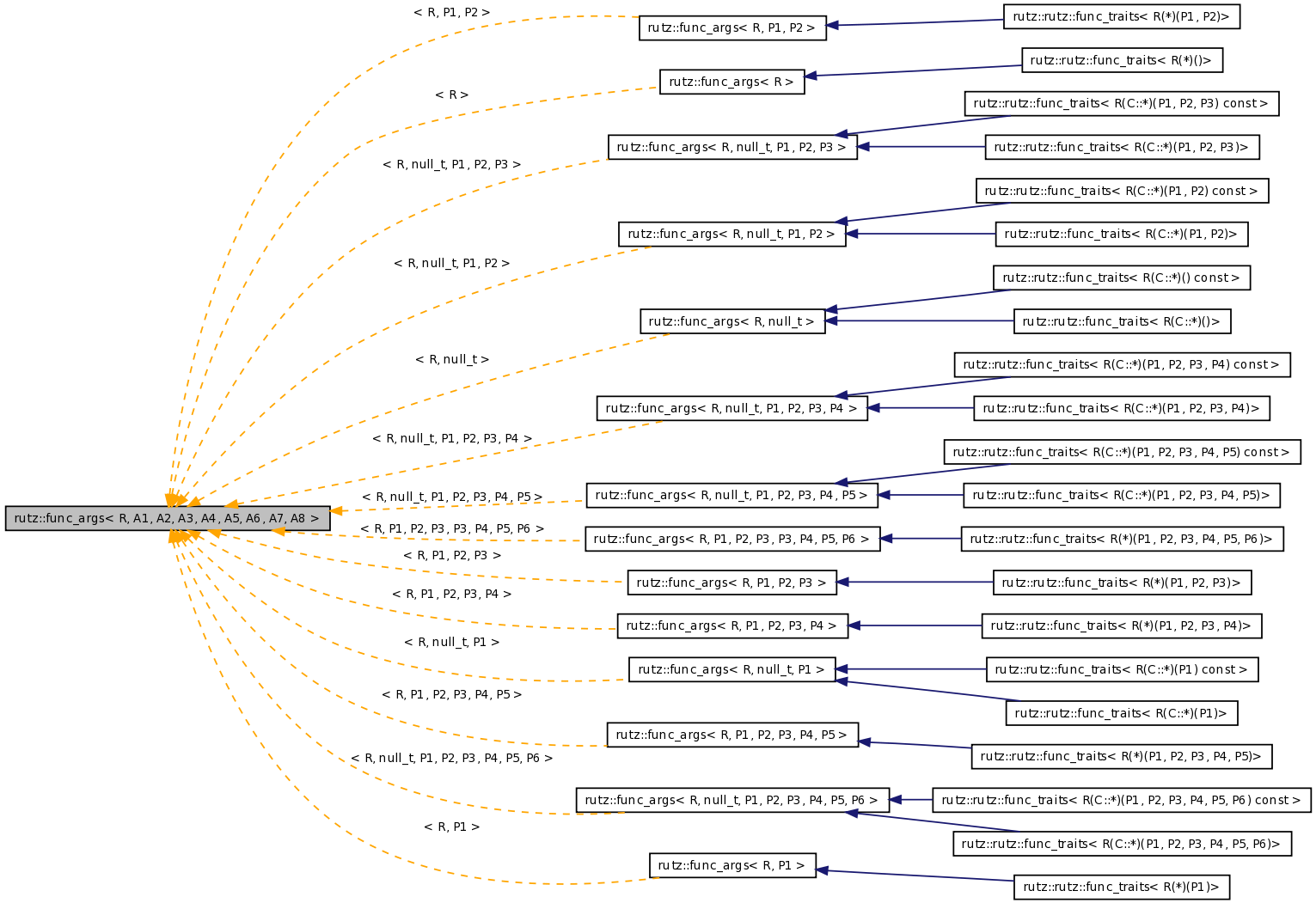# rutz::func_args< R, A1, A2, A3, A4, A5, A6, A7, A8 > Struct Template Reference

Holds typedefs for the types of a function's arguments and return value. More...

`#include <rutz/functors.h>`

Inheritance diagram for rutz::func_args< R, A1, A2, A3, A4, A5, A6, A7, A8 >:[legend]

List of all members.

## Public Types

typedef R retn_t
typedef A1 arg1_t
typedef A2 arg2_t
typedef A3 arg3_t
typedef A4 arg4_t
typedef A5 arg5_t
typedef A6 arg6_t
typedef A7 arg7_t
typedef A8 arg8_t

## Detailed Description

### template<class R = void, class A1 = null_t, class A2 = null_t, class A3 = null_t, class A4 = null_t, class A5 = null_t, class A6 = null_t, class A7 = null_t, class A8 = null_t> struct rutz::func_args< R, A1, A2, A3, A4, A5, A6, A7, A8 >

Holds typedefs for the types of a function's arguments and return value.

Definition at line 54 of file functors.h.

The documentation for this struct was generated from the following file:

The software described here is Copyright (c) 1998-2005, Rob Peters.
This page was generated Wed Dec 3 06:56:35 2008 by Doxygen version 1.5.5.